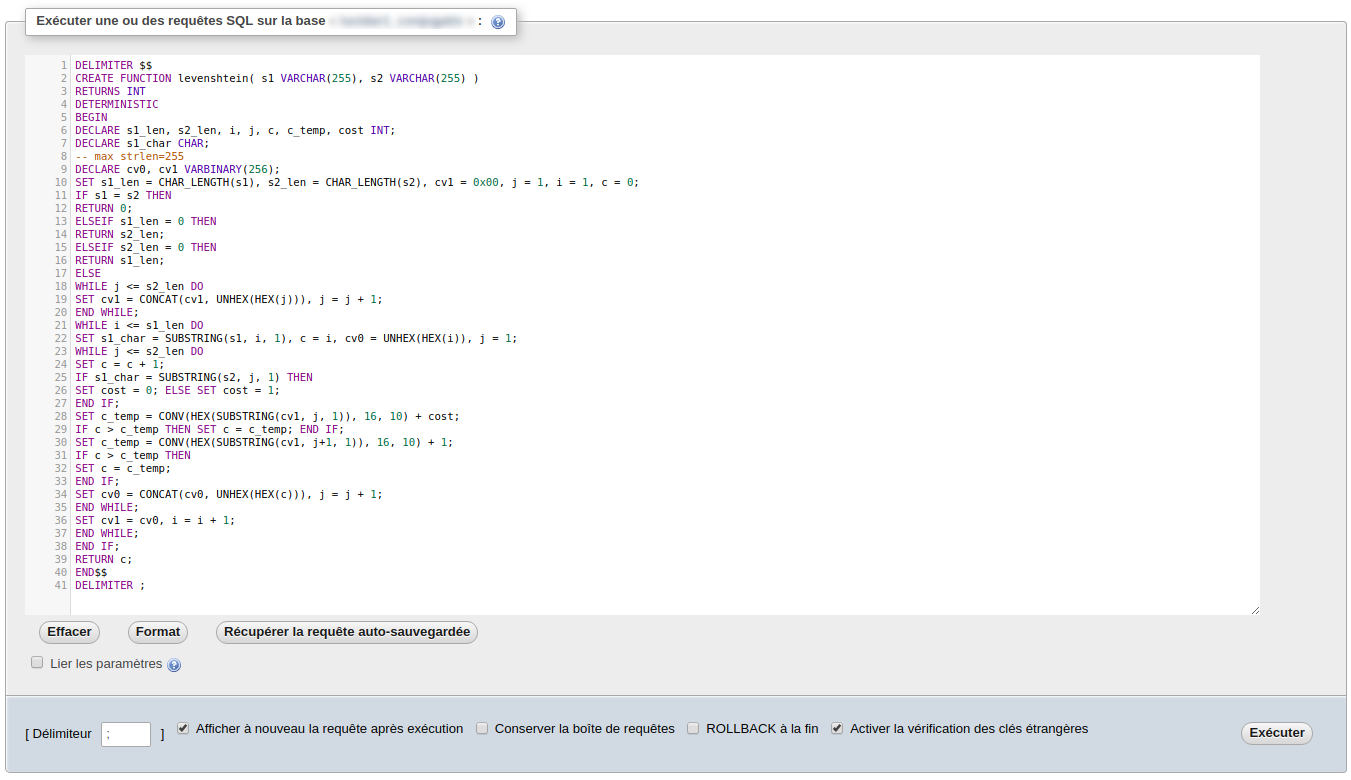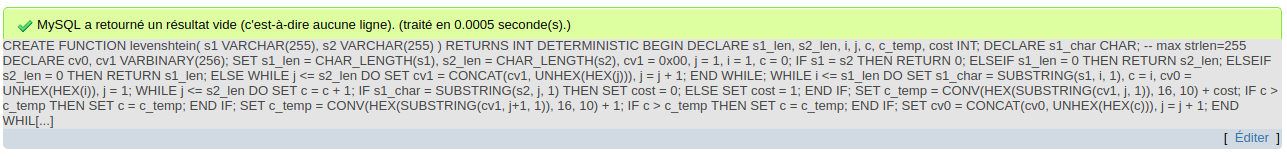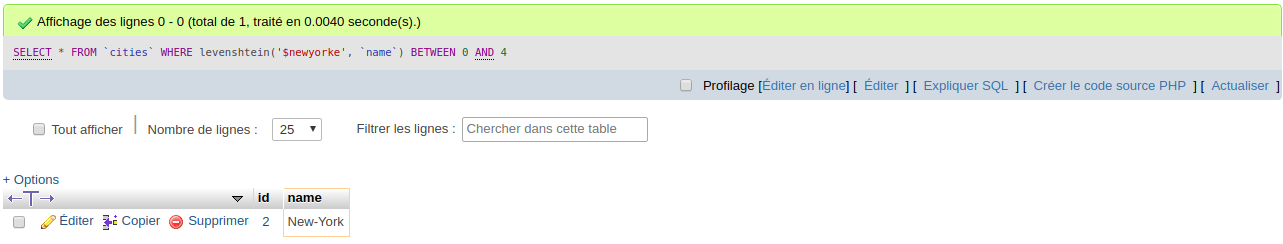Do me a favor, take a few seconds to have a look at my last project.

Thank you, Lulu

# Levenshtein distance in mySQL

## Introduction

According to Wikipedia, the Levenshtein distance is a string metric for measuring the difference between two sequences. Informally, the Levenshtein distance between two words is the minimum number of single-character edits (insertions, deletions or substitutions) required to change one word into the other. This distance can be used to find a row in a SQL database where the keyword does not match exactly the fields. By computing the distance between the keywords and the candidates, it becomes possible to find the closest (in term of Levenshtein distance) row in the database. It can be usefull for example for finding a nickname, a username or a city even if the keywords does not match exactly.

Let's take an example. In the following, we'll assume the database contains a row containing the field "New-York". The user was asked for a city and input the following:

User input Database Distance Comment
New-York New-York 0 The words match perfectly, the distance is 0.
New York New-York 1 One substitution.
NewYork New-York 1 One insertion.
New-Yorke New-York 1 One deletion.
NewYorke New-York 2 One insertion and one deletion.
Paris New-York 8 Five substitutions and three deletions.

The following implementation has been tested with these versions:

• SQL 5.5.57-0 / ubuntu0.14.04.1
• SQL 5.6.39-cll-lve

## Implementation of the function

The first step is to implement the levenshtein function in mySQL. Select the table where the distance between words is required and copy the following SQL code (from http://www.artfulsoftware.com):

DELIMITER $$CREATE FUNCTION levenshtein( s1 VARCHAR(255), s2 VARCHAR(255) ) RETURNS INT DETERMINISTIC BEGIN DECLARE s1_len, s2_len, i, j, c, c_temp, cost INT; DECLARE s1_char CHAR; -- max strlen=255 DECLARE cv0, cv1 VARBINARY(256); SET s1_len = CHAR_LENGTH(s1), s2_len = CHAR_LENGTH(s2), cv1 = 0x00, j = 1, i = 1, c = 0; IF s1 = s2 THEN RETURN 0; ELSEIF s1_len = 0 THEN RETURN s2_len; ELSEIF s2_len = 0 THEN RETURN s1_len; ELSE WHILE j <= s2_len DO SET cv1 = CONCAT(cv1, UNHEX(HEX(j))), j = j + 1; END WHILE; WHILE i <= s1_len DO SET s1_char = SUBSTRING(s1, i, 1), c = i, cv0 = UNHEX(HEX(i)), j = 1; WHILE j <= s2_len DO SET c = c + 1; IF s1_char = SUBSTRING(s2, j, 1) THEN SET cost = 0; ELSE SET cost = 1; END IF; SET c_temp = CONV(HEX(SUBSTRING(cv1, j, 1)), 16, 10) + cost; IF c > c_temp THEN SET c = c_temp; END IF; SET c_temp = CONV(HEX(SUBSTRING(cv1, j+1, 1)), 16, 10) + 1; IF c > c_temp THEN SET c = c_temp; END IF; SET cv0 = CONCAT(cv0, UNHEX(HEX(c))), j = j + 1; END WHILE; SET cv1 = cv0, i = i + 1; END WHILE; END IF; RETURN c; END$$
DELIMITER ;

Run the query on your server.Result must be OK before continuing:The function must appear in your current table. It may need few minutes to be refreshed, depending on your version. Refresh the whole page if needed.## Call the function

Before performing complex queries, let's check that the function works properly. Run the following query:

SELECT levenshtein('New-York', 'Paris')

It should return a distance of 8:The Levenshtein function can now be used in a SQL query:

SELECT * FROM cities WHERE levenshtein('\$keyword', name) BETWEEN 0 AND 4

The previous query returns the rows of table cities where the distance between the string keyword and the field name is less than 5. Let's try with the word newyorke:That's all folks !

## See also

Last update : 04/13/2019